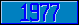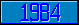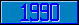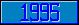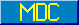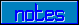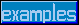## \$%SQRT

### M[UMPS] by Example

```
2-Jul–96, 6:54:35

Routine Save for General Mathematics Library function \$%SQRT^MATH

;

; Unless otherwise noted, the code below

; was approved in document X11/95–11

;

; If corrections have been applied,

; first the original line appears,

; with three semicolons at the beginning of the line.

;

; Then the source of the correction is acknowledged,

; then the corrected line appears, followed by a

; line containing three semicolons.

;

SQRT(X,PREC) ;

If X<0 Set \$Ecode=",M28,"

If X=0 Quit 0

;

;;;

; Alan Frank (October 1995)

Set PREC=\$Get(PREC,11)

;;;

;

;

;;; If X<1 Quit 1/\$%SQRT^MATH(1/X)

; Winfried Gerum (8 June 1995)

If X<1 Quit 1/\$%SQRT^MATH(1/X,PREC)

;;;

;

New P,R,E

Set PREC=\$Get(PREC,11)+1

;

;;; Set @(E="1E-"_PREC)

; Winfried Gerum (8 June 1995)

; Eli Reidler (28 June 1996)

Set @("E=1E-"_PREC)

;;;

;

Set R=X

For  Set P=R,R=X/R+R/2,P=P-R/R If -E<P,P<E Quit

Quit R

;===

;

;

;

```